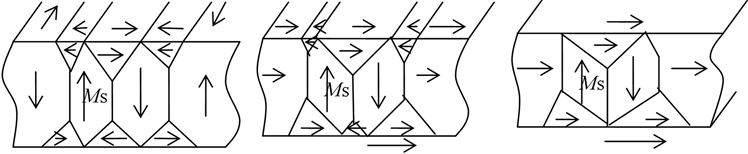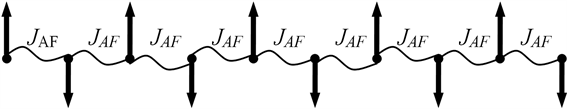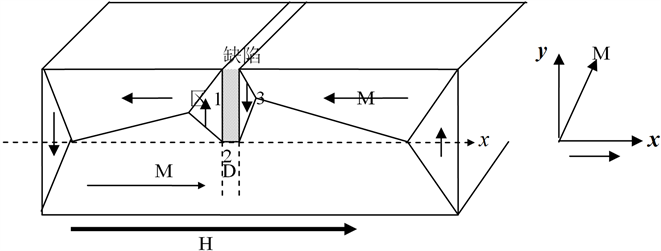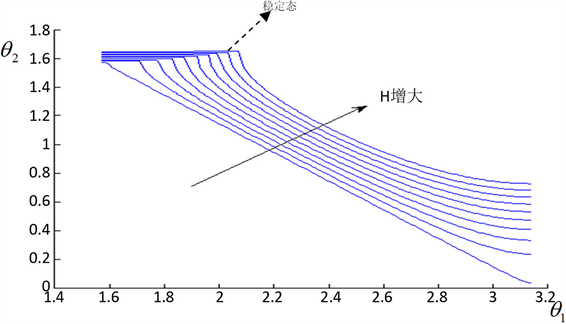﻿ 基于微磁学的损伤缺陷检测研究

# 基于微磁学的损伤缺陷检测研究Micromagnetic Analysis on Leakage Magnetic Field of Defects

Abstract: The change included the domain structure inside the ferromagnetic materials and two side of the defect and the energy of the domain walls were analyzed. It can be concluded that the defect in the ferromagnetic materials can fix the domain wall; the reverse potential wall in the side of the defect blocks the movement of the domain walls; then the micromagnetic fixed knot comes into being around the defect. The direction of the magnetization whose normal component is discontinuous in the micromagnetic fixed knot deciding the character of the flux magnetic field which contains the information of the defect. By testing this flux magnetic field, we can realize the defect testing.

1. 引言

2. 铁磁物质的磁化

3. 缺陷材料的磁化Figure 1. The change of the domain under external magnetic field

$E=-\underset{ij}{\sum }{J}_{ij}\left({S}_{i}\cdot {S}_{j}\right)-\underset{i}{\sum }\left(H\cdot {S}_{i}\right)$ (1)Figure 2. Atomic Spin and its energy in the Ferromagnetic Material

${E}_{0}=-9{J}_{AF}×\left(-1\right)$ (2)

${E}_{1}=-2{J}_{F}×1-7{J}_{AF}×\left(-1\right)-2H$ (3)

${E}_{1}-{E}_{0}=-2{J}_{F}-2{J}_{AF}-2H<0$ (4)

$H>-2{J}_{F}-2{J}_{AF}$

4. 缺陷对畴壁的钉扎Figure 3. The pin of domain wall on defects

5. 畴壁钉扎下缺陷两侧磁化方向的变化

$F=\int \left[{A}_{i}{\left(\frac{\text{d}\theta }{\text{d}x}\right)}^{2}+{K}_{i}{\mathrm{sin}}^{2}\theta -{\mu }_{0}H{M}_{i}\mathrm{cos}\theta \right]\text{d}x$ (5)

$-2{A}_{i}\frac{{\text{d}}^{2}\theta }{\text{d}{x}^{2}}+2{K}_{i}\mathrm{sin}\theta \mathrm{cos}\theta +{\mu }_{0}H{M}_{i}\mathrm{sin}\theta =0$ (6)

$-{A}_{i}{\left(\frac{\text{d}\theta }{\text{d}x}\right)}^{2}+{K}_{i}{\mathrm{sin}}^{2}\theta -{\mu }_{0}H{M}_{i}\mathrm{cos}\theta ={C}_{i}$ (7)

$i=1,2,3$ 分别表示图3中的区域1，2，3。Ci为积分常数，在区域1，2，3有不同的值。在1，3均匀区的边界条件为：

$\begin{array}{l}\theta \left(x=-\infty \right)=0,\theta \left(x=+\infty \right)=\pi \\ {\left(\frac{\text{d}\theta }{\text{d}x}\right)}_{x=-\infty }=0,{\left(\frac{\text{d}\theta }{\text{d}x}\right)}_{x=+\infty }=0\end{array}\right\}$ (8)

${C}_{1}=-{\mu }_{0}H{M}_{1},{C}_{3}={\mu }_{0}H{M}_{1}$

$\begin{array}{l}-{A}_{1}{\left(\frac{\text{d}\theta }{\text{d}x}\right)}^{2}+{K}_{1}{\mathrm{sin}}^{2}\theta -{\mu }_{0}H{M}_{1}\mathrm{cos}\theta +{\mu }_{0}H{M}_{1}=0\\ -{A}_{1}{\left(\frac{\text{d}\theta }{\text{d}x}\right)}^{2}+{K}_{1}{\mathrm{sin}}^{2}\theta -{\mu }_{0}H{M}_{1}\mathrm{cos}\theta -{\mu }_{0}H{M}_{1}=0\end{array}$ (9)

$-{A}_{2}{\left(\frac{\text{d}\theta }{\text{d}x}\right)}^{2}+{K}_{2}{\mathrm{sin}}^{2}\theta -{\mu }_{0}H{M}_{2}\mathrm{cos}\theta ={C}_{2}$ (10)

$\begin{array}{l}{A}_{1}{\frac{\text{d}\theta }{\text{d}x}|}_{x={x}_{1}}=A{\frac{\text{d}\theta }{\text{d}x}|}_{x={x}_{1}}\\ {A}_{1}{\frac{\text{d}\theta }{\text{d}x}|}_{x={x}_{2}}={A}_{2}{\frac{\text{d}\theta }{\text{d}x}|}_{x={x}_{21}}\end{array}$ (11)

$h=\frac{{\mu }_{0}H{M}_{1}}{{K}_{1}},a=1-\frac{{M}_{2}{A}_{2}}{{M}_{1}{A}_{1}},b=1-\frac{{A}_{2}{K}_{2}}{{A}_{1}{K}_{1}}$，根据上述连续性条件，可消去2区中的积分常数C2，得到：

${\left(\mathrm{cos}{\theta }_{1}+\frac{ha}{2b}\right)}^{2}-{\left(\mathrm{cos}{\theta }_{2}+\frac{ha}{2b}\right)}^{2}=\frac{2h}{b}$ (12)

${K}_{1}=4.8×{10}^{5}\text{erg}/{\text{cm}}^{3}$${M}_{1}=1707G\left(290K\right)$。则由式 $h=\frac{{\mu }_{0}H{M}_{1}}{{K}_{1}}$ 可计算得h，根据式(12)，作出 ${\theta }_{1}$${\theta }_{2}$ 随外磁场H不断增大时二者之间的变化曲线，如图4所示。Figure 4. The relation of magnetization direction at two side of pinned domain wall

6. 结论

 冷建成, 刘扬, 周国强, 吴泽民, 闫天红. 铁磁性材料早期损伤的磁无损检测方法综述[J]. 化工机械, 2013, 40(2): 139-145.

 张轩睿. 基于磁性技术的无损检测方法研究[J]. 商品与质量, 2016(27): 206.

 李冲冲, 董丽虹, 王海斗. 疲劳损伤磁性无损评估技术研究现状及发展前景[J]. 材料导报, 2015(15): 107-113.

 任吉林, 林俊明, 等, 著. 金属磁记忆检测技术[M]. 北京: 中国电力出版社, 2000: 20-50.

 葛静. 磁记忆检测技术[J]. 建筑工程技术与设计, 2018(25): 405.

 马惠香, 周建庭, 等. 基于金属磁记忆技术的钢筋应力无损检测试验[J]. 江苏大学学报(自然科学版), 2018, 39(3): 349-354.

 杨茂, 周建庭, 等. 混凝土内部钢筋锈蚀的磁记忆检测[J]. 建筑材料学报, 2018, 21(2): 345-350.

 姜寿亭, 李卫, 编著. 凝聚态磁性物理[M]. 北京: 科学出版社, 2003: 1-100.

 钟文定. 铁磁学(中册) [M]. 北京: 科学出版社, 2000: 1-120.

 奥汉德利. 现代磁性材料原理和应用[M]. 北京: 化学工业出版社, 2002: 20-60.

 仲维畅. 方钢纵向磁化时各棱上的磁荷线密度[J]. 无损检测, 2002, 24(8): 332-335.

 田民波. 磁性材料[M]. 北京: 清华大学出版社, 2001: 1-120.

 祖瑞丽, 任尚坤, 等. 基于微磁学的金属磁记忆检测机理分析[J]. 南昌航空大学学报(自然科学版), 2017, 31(3): 34-39.

 徐章遂, 等. 裂纹漏磁定量检测原理与应用[M]. 北京: 国防工业出版社, 2005: 22-24.

Top